cancel
Showing results for
Did you mean:

No ratings

When using ADCs it is not enough to just configure the module, add a clock signal, apply the Nyquist criteria and hope for the best, because normally that is just not enough. Even if we use the best software configuration, sampling rate, conversion time, etc; we might end up with noisy conversions, and worst of all a low ENOB figure which sums up in a lousy, low resolution ADC application. To complement the software end you need to follow some basic hardware design rules, some of them might seem logical, other might even weird or excessive however they are the key to a successful conversion, I took the time to compile a short list of effective design best practices trying to cover the basics of ADC design. If you think I missed something feel free to comment and ask for more information.

Ground Isolation

Because ground is the power return for all digital circuits and analog circuits, one of the most basic design philosophies is to isolate digital and analog grounds. If the grounds are not isolated, the return from the analog circuitry will flow through the analog ground impedance and the digital ground current will flow through the analog ground, usually the digital ground current is typically much greater than the analog ground current.  As the frequency of digital circuits increases, the noise generated on the ground increases dramatically. CMOS logic families are of the saturating type; this means the logic transitions cause large transient currents on the power supply and ground. CMOS outputs connect the power to ground through a low impedance channel during the logic transitions. Digital logic waveforms are rectangular waves which imply many higher frequency harmonic components are induced by high speed transmission lines and clock signals.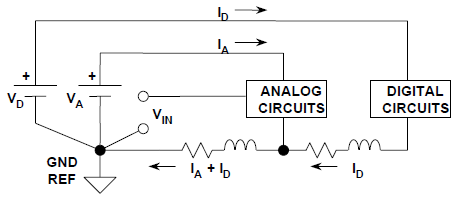Figure 1: Typical mixed signal circuit grounding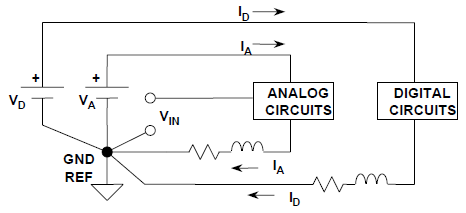Figure 2: Isolated mixed signal circuit grounding

Inductive decoupling

Another potential problem is the coupling of signal from one circuit to another via mutual inductance and it does not matter if you think the signals are too weak to have a real effect, the amount of coupling will depend on the strength of the interference, the mutual inductance, the area enclosed by the signal loop (which is basically an antenna), and the frequency. It will also depend primarily on the physical proximity of the loops, as well as the permeability of the material. This inductive coupling is also known as crosstalk in data lines.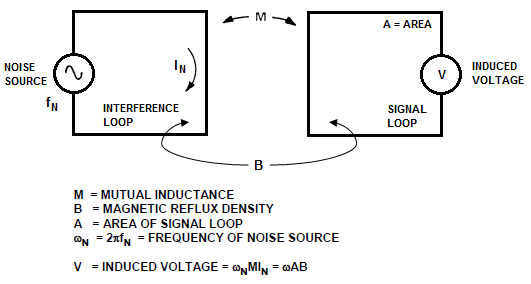Figure 3: Coupling induced noise

It may seem logical to use a single trace as the return path for the two sources (dotted lines). However, this would cause the return currents for both signals to flow through the same impedance, in addition; it will maximize the area of the interference loops and increase the mutual inductance by moving the loops close together. This will increase the mutual noise inductance and the coupling between the circuits. Routing the traces in the manner shown below minimizes the area enclosed by the loops and separates the return paths, thus separating the circuits and, in turn, minimizing the mutual noise inductance.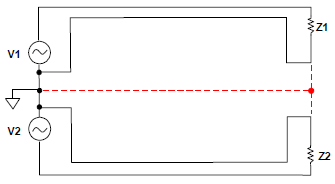Figure 4: Inductance decoupling layout

Power supply decoupling

The idea after power decoupling is to create a low noise environment for the analog circuitry to operate. In any given circuit the power supply pin is really in series with the output, therefore, any high frequency energy on the power line will couple to the output directly, which makes it necessary to keep this high frequency energy from entering the analog circuitry. This is done by using a small capacitor to short the high frequency signals away from the chip to the circuit’s ground line. A disadvantage of high frequency decoupling is it makes a circuit more prone to low frequency noise however it is easily solved by adding a larger capacitor.

Optimal power supply decoupling

• A large electrolytic capacitor (10 μF – 100 μF) no more than 2 in. away from the chip.
• A small capacitor (0.01 μF – 0.1 μF) as close to the power pins of the chip as possible.
• A small ferrite bead in series with the supply pin (Optional).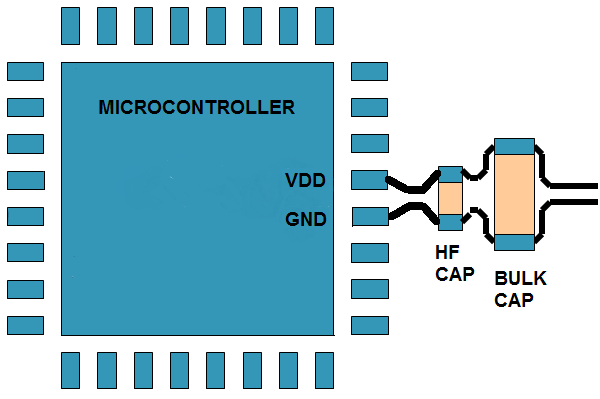Figure 5: Power supply decoupling layout

Treat signal lines as transmission lines

Although signal coupling can be minimized it cannot be avoided, the best approach to effectively counteract its effects on signal lines is to channel it into a conductor of our choice, in this case the circuit’s ground is the best choice to channel the effects of inductive coupling; we can accomplish this by routing ground lines along signal lines as close as manufacturing capabilities allow. An very effective way to accomplish this is routing signals in triplets, these works for both digital and analog signals.The advantages of doing so are an improved immunity not only to inductive coupling but also immunity to external noise.

Optimal routing:

• Routing in “triplets” (S-G-S) provide good signal coupling with relatively low impact on routing density
• Ground trace needs to be connected to the ground pins on the source and destination devices for the signal traces
• Spacing should be as close as manufacturing will allow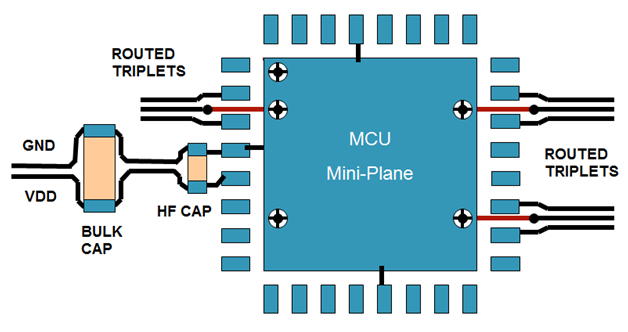Figure 6: Transmission line routing

Signal acquisition circuit

To improve noise immunity an external RC acquisition circuit can be added to the ADC input, it consists of a resistor in series with the ADC input and a capacitor going from the input to the circuit’s ground as the figure below shows: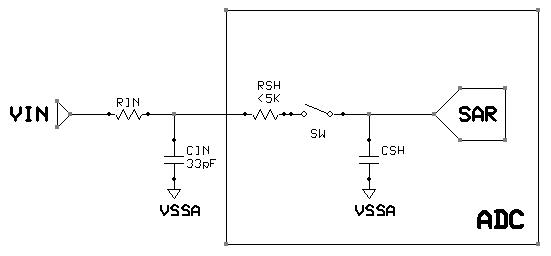Figure 7: ADC with an external acquisition circuit

The external RC circuit values depend on the internal characteristics and configuration of the ADC you use, such as the availability of an internal gain amplifier or the ADC’s architecture; the equation and circuit shown here represents a simplified form of ADC used in Freescale devices. The equivalent sampling resistance RSH is represented by total serial resistance connected between sampling capacitance and analog input pin (sampling switch, multiplexor switches etc.). The sampling capacitance CSH is represented by total parallel capacitance. For example in a case of Freescale SAR ADC equivalent sampling capacitance contains bank of capacitances. The equation shown how to calculate the value of the input resistor based on the values of both the input and sample and hold circuit.

It must be noted the mentioned figures could have an alternate designation in any given datasheet; the ones mentioned here are specific to Kinetis devices:

CIN=       Input capacitance (33pF min)

CSH=      Sample & Hold circuit capacitance ( CDAIN in datasheet)

VIN=       Input voltage level

VCSH0= Initial voltage across S&H circuit (0V)

VSFR=    Full scale voltage (VDDA)

N=           bit resolution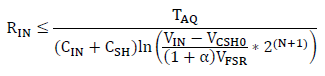Note:  Special care must be taken when performing the calculation since a deviation from the correct values will result in a significant conversion error due to signal distortion.

Labels (5)

• ### Kinetis L Series MCUs

Hi,

What is the units of time (us, ms or second) and capacitance (pF, uF, mF or F) in this calculation?

And also what is the alfa value in this formula?